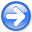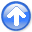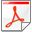Efficient depth extrapolation of waves in elastic isotropic mediaNext: The Method Up: Maharramov: Efficient elastic modeling Previous: Maharramov: Efficient elastic modeling

# Introduction

Extrapolation of seismic wave fields in depth using one-way propagation operators is an efficient alternative to time- and frequency-domain modeling with the full wave equation, particularly in seismic migration applications (see (Claerbout, 1985), (Biondi, 2005)). While one-way extrapolators have long been established as key components of the seismic imaging toolbox for isotropic acoustic media, extrapolation of elastic wave fields is still carried out by solving the full elastodynamic system either in the time or frequency domain, either approach being computationally expensive. The high computational cost of wave extrapolation in elastic media is one of the barriers to a widespread adoption of multicomponent seismic processing in industrial applications. Some progress has been made recently in the development of efficient one-way methods for certain simplest anisotropic elastic models (e.g., vertically transversally isotropic or tilted transversally isotropic media - see (Shan, 2007), (Nolte, 2008), (Maharramov and Nolte, 2011)) However, these methods use the ``pseudoacoustic'' approximation (see (Grechka, 2009)) and are used for a kinematically accurate propagation of pressure waves only.

In this paper we present a method for one-way frequency-domain extrapolation of displacement fields in an elastic isotropic medium. The approach of this paper is based on factorizing the elastic wave equation using pseudo-differential operators without introducing stress-related unknown functions into the equations. Our approach is conceptually similar to the derivation of the acoustic single square-root equation (see (Claerbout, 1985)), except the resulting factorized propagation operators can not be obtained analytically, but are computed numerically.Efficient depth extrapolation of waves in elastic isotropic mediaNext: The Method Up: Maharramov: Efficient elastic modeling Previous: Maharramov: Efficient elastic modeling

2012-10-29